# How to drop one or multiple columns in Pandas Dataframe

Let’s discuss how to drop one or multiple columns in Pandas Dataframe. Drop one or more than one columns from a DataFrame can be achieved in multiple ways.

Create a simple dataframe with dictionary of lists, say column names are A, B, C, D, E.

 `# Import pandas package  ` `import` `pandas as pd ` ` `  `# create a dictionary with five fields each ` `data ``=` `{ ` `    ``'A'``:[``'A1'``, ``'A2'``, ``'A3'``, ``'A4'``, ``'A5'``],  ` `    ``'B'``:[``'B1'``, ``'B2'``, ``'B3'``, ``'B4'``, ``'B5'``],  ` `    ``'C'``:[``'C1'``, ``'C2'``, ``'C3'``, ``'C4'``, ``'C5'``],  ` `    ``'D'``:[``'D1'``, ``'D2'``, ``'D3'``, ``'D4'``, ``'D5'``],  ` `    ``'E'``:[``'E1'``, ``'E2'``, ``'E3'``, ``'E4'``, ``'E5'``] } ` ` `  `# Convert the dictionary into DataFrame  ` `df ``=` `pd.DataFrame(data) ` ` `  `df `

Output: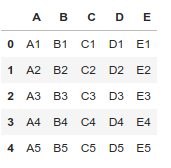Method #1: Drop Columns from a Dataframe using `drop()` method.

Remove specific single column.

 `# Import pandas package  ` `import` `pandas as pd ` ` `  `# create a dictionary with five fields each  ` `data ``=` `{ ` `    ``'A'``:[``'A1'``, ``'A2'``, ``'A3'``, ``'A4'``, ``'A5'``],  ` `    ``'B'``:[``'B1'``, ``'B2'``, ``'B3'``, ``'B4'``, ``'B5'``],  ` `    ``'C'``:[``'C1'``, ``'C2'``, ``'C3'``, ``'C4'``, ``'C5'``],  ` `    ``'D'``:[``'D1'``, ``'D2'``, ``'D3'``, ``'D4'``, ``'D5'``],  ` `    ``'E'``:[``'E1'``, ``'E2'``, ``'E3'``, ``'E4'``, ``'E5'``] } ` ` `  `# Convert the dictionary into DataFrame  ` `df ``=` `pd.DataFrame(data) ` ` `  `# Remove column name 'A' ` `df.drop([``'A'``], axis ``=` `1``) `

Output: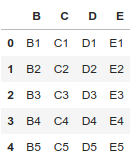Remove specific multiple columns.

 `# Import pandas package  ` `import` `pandas as pd ` ` `  `# create a dictionary with five fields each ` `data ``=` `{ ` `    ``'A'``:[``'A1'``, ``'A2'``, ``'A3'``, ``'A4'``, ``'A5'``],  ` `    ``'B'``:[``'B1'``, ``'B2'``, ``'B3'``, ``'B4'``, ``'B5'``],  ` `    ``'C'``:[``'C1'``, ``'C2'``, ``'C3'``, ``'C4'``, ``'C5'``],  ` `    ``'D'``:[``'D1'``, ``'D2'``, ``'D3'``, ``'D4'``, ``'D5'``],  ` `    ``'E'``:[``'E1'``, ``'E2'``, ``'E3'``, ``'E4'``, ``'E5'``] } ` ` `  `# Convert the dictionary into DataFrame  ` `df ``=` `pd.DataFrame(data) ` ` `  `# Remove two columns name is 'C' and 'D' ` `df.drop([``'C'``, ``'D'``], axis ``=` `1``) ` ` `  `# df.drop(columns =['C', 'D']) `

Output: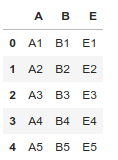Remove columns as based on column index.

 `# Import pandas package  ` `import` `pandas as pd ` ` `  `# create a dictionary with five fields each ` `data ``=` `{ ` `    ``'A'``:[``'A1'``, ``'A2'``, ``'A3'``, ``'A4'``, ``'A5'``],  ` `    ``'B'``:[``'B1'``, ``'B2'``, ``'B3'``, ``'B4'``, ``'B5'``],  ` `    ``'C'``:[``'C1'``, ``'C2'``, ``'C3'``, ``'C4'``, ``'C5'``],  ` `    ``'D'``:[``'D1'``, ``'D2'``, ``'D3'``, ``'D4'``, ``'D5'``],  ` `    ``'E'``:[``'E1'``, ``'E2'``, ``'E3'``, ``'E4'``, ``'E5'``] } ` ` `  `# Convert the dictionary into DataFrame  ` `df ``=` `pd.DataFrame(data) ` ` `  `# Remove three columns as index base ` `df.drop(df.columns[[``0``, ``4``, ``2``]], axis ``=` `1``, inplace ``=` `True``) ` ` `  `df `

Output: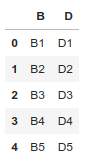Method #2: Drop Columns from a Dataframe using `iloc[]` and `drop()` method.

Remove all columns between a specific column to another columns.

 `# Import pandas package  ` `import` `pandas as pd ` `# create a dictionary with five fields each  ` `data ``=` `{ ` `    ``'A'``:[``'A1'``, ``'A2'``, ``'A3'``, ``'A4'``, ``'A5'``],  ` `    ``'B'``:[``'B1'``, ``'B2'``, ``'B3'``, ``'B4'``, ``'B5'``],  ` `    ``'C'``:[``'C1'``, ``'C2'``, ``'C3'``, ``'C4'``, ``'C5'``],  ` `    ``'D'``:[``'D1'``, ``'D2'``, ``'D3'``, ``'D4'``, ``'D5'``],  ` `    ``'E'``:[``'E1'``, ``'E2'``, ``'E3'``, ``'E4'``, ``'E5'``] } ` ` `  `# Convert the dictionary into DataFrame  ` `df ``=` `pd.DataFrame(data) ` ` `  `# Remove all columns between column index 1 to 3 ` `df.drop(df.iloc[:, ``1``:``3``], inplace ``=` `True``, axis ``=` `1``) ` ` `  `df `

Output: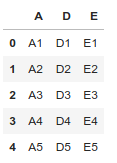Method #3: Drop Columns from a Dataframe using `ix()` and `drop()` method.

Remove all columns between a specific column name to another columns name.

 `# Import pandas package  ` `import` `pandas as pd ` ` `  `# create a dictionary with five fields each  ` `data ``=` `{ ` `    ``'A'``:[``'A1'``, ``'A2'``, ``'A3'``, ``'A4'``, ``'A5'``],  ` `    ``'B'``:[``'B1'``, ``'B2'``, ``'B3'``, ``'B4'``, ``'B5'``],  ` `    ``'C'``:[``'C1'``, ``'C2'``, ``'C3'``, ``'C4'``, ``'C5'``],  ` `    ``'D'``:[``'D1'``, ``'D2'``, ``'D3'``, ``'D4'``, ``'D5'``],  ` `    ``'E'``:[``'E1'``, ``'E2'``, ``'E3'``, ``'E4'``, ``'E5'``] } ` ` `  `# Convert the dictionary into DataFrame  ` `df ``=` `pd.DataFrame(data) ` ` `  `# Remove all columns between column name 'B' to 'D' ` `df.drop(df.ix[:, ``'B'``:``'D'``].columns, axis ``=` `1``) `

Output: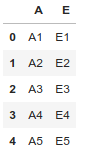Method #4: Drop Columns from a Dataframe using `loc[]` and `drop()` method.

Remove all columns between a specific column name to another columns name.

 `# Import pandas package  ` `import` `pandas as pd ` ` `  `# create a dictionary with five fields each  ` `data ``=` `{ ` `    ``'A'``:[``'A1'``, ``'A2'``, ``'A3'``, ``'A4'``, ``'A5'``],  ` `    ``'B'``:[``'B1'``, ``'B2'``, ``'B3'``, ``'B4'``, ``'B5'``],  ` `    ``'C'``:[``'C1'``, ``'C2'``, ``'C3'``, ``'C4'``, ``'C5'``],  ` `    ``'D'``:[``'D1'``, ``'D2'``, ``'D3'``, ``'D4'``, ``'D5'``],  ` `    ``'E'``:[``'E1'``, ``'E2'``, ``'E3'``, ``'E4'``, ``'E5'``] } ` ` `  `# Convert the dictionary into DataFrame  ` `df ``=` `pd.DataFrame(data) ` ` `  `# Remove all columns between column name 'B' to 'D' ` `df.drop(df.loc[:, ``'B'``:``'D'``].columns, axis ``=` `1``) `

Output: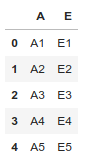Note: Different `loc()` and `iloc()` is `iloc()` exclude last column range element.

Method #5: Drop Columns from a Dataframe by iterative way.

Remove all columns between a specific column name to another columns name.

 `# Import pandas package  ` `import` `pandas as pd ` ` `  `# create a dictionary with five fields each  ` `data ``=` `{ ` `    ``'A'``:[``'A1'``, ``'A2'``, ``'A3'``, ``'A4'``, ``'A5'``],  ` `    ``'B'``:[``'B1'``, ``'B2'``, ``'B3'``, ``'B4'``, ``'B5'``],  ` `    ``'C'``:[``'C1'``, ``'C2'``, ``'C3'``, ``'C4'``, ``'C5'``],  ` `    ``'D'``:[``'D1'``, ``'D2'``, ``'D3'``, ``'D4'``, ``'D5'``],  ` `    ``'E'``:[``'E1'``, ``'E2'``, ``'E3'``, ``'E4'``, ``'E5'``] } ` ` `  `# Convert the dictionary into DataFrame  ` `df ``=` `pd.DataFrame(data) ` `for` `col ``in` `df.columns: ` `    ``if` `'A'` `in` `col: ` `        ``del` `df[col] ` ` `  `df `

Output: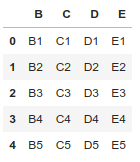My Personal Notes arrow_drop_upCheck out this Author's contributed articles.

If you like GeeksforGeeks and would like to contribute, you can also write an article using contribute.geeksforgeeks.org or mail your article to contribute@geeksforgeeks.org. See your article appearing on the GeeksforGeeks main page and help other Geeks.

Please Improve this article if you find anything incorrect by clicking on the "Improve Article" button below.

Article Tags :

2

Please write to us at contribute@geeksforgeeks.org to report any issue with the above content.